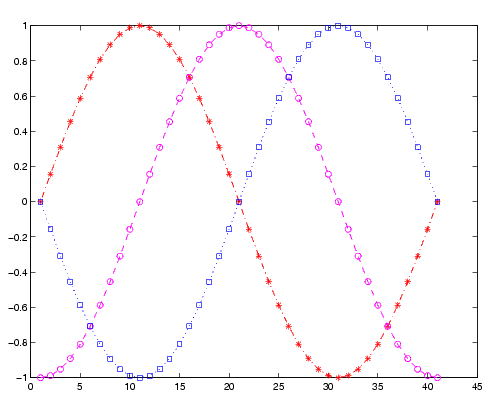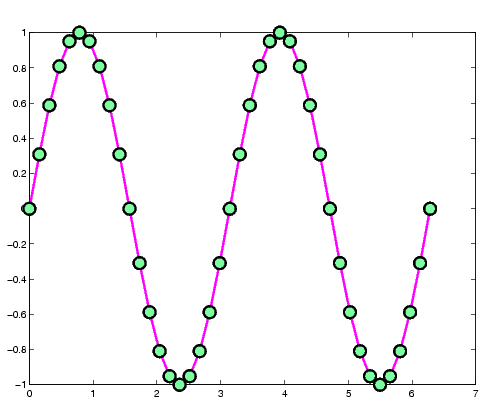MATLAB Function ReferenceLineSpec

Line specification syntax

Description

This page describes how to specify the properties of lines used for plotting. MATLAB enables you to define many characteristics, including

• Line style
• Line width
• Color
• Marker type
• Marker size
• Marker face and edge coloring (for filled markers)

MATLAB defines string specifiers for line styles, marker types, and colors. The following tables list these specifiers.

Line Style Specifiers

 Specifier Line Style `- ` Solid line (default) `--` Dashed line `:` Dotted line `-. ` Dash-dot line

Marker Specifiers

 Specifier Marker Type `+` Plus sign `o` Circle `*` Asterisk `.` Point `x` Cross `'square' or s` Square `'diamond' or d` Diamond `^` Upward-pointing triangle `v` Downward-pointing triangle `>` Right-pointing triangle `<` Left-pointing triangle `'pentagram' or p` Five-pointed star (pentagram) `'hexagram' or h` Six-pointed star (hexagram)

Color Specifiers

 Specifier Color `r` Red `g` Green `b` Blue `c` Cyan `m` Magenta `y` Yellow `k` Black `w` White

Many plotting commands accept a `LineSpec` argument that defines three components used to specify lines:

• Line style
• Marker symbol
• Color

For example,

• ```plot(x,y,'-.or')
```

plots `y` versus `x` using a dash-dot line (-`.`), places circular markers (`o`) at the data points, and colors both line and marker red (`r`). Specify the components (in any order) as a quoted string after the data arguments.

Plotting Data Points with No Line

If you specify a marker, but not a line style, MATLAB plots only the markers. For example,

• ```plot(x,y,'d')
```

Related Properties

When using the `plot` and `plot3` functions, you can also specify other characteristics of lines using graphics properties:

• `LineWidth` -- Specifies the width (in points) of the line
• `MarkerEdgeColor` -- Specifies the color of the marker or the edge color for filled markers (circle, square, diamond, pentagram, hexagram, and the four triangles)
• `MarkerFaceColor` -- Specifies the color of the face of filled markers
• `MarkerSize` -- Specifies the size of the marker in points

In addition, you can specify the `LineStyle`, `Color`, and `Marker` properties instead of using the symbol string. This is useful if you want to specify a color that is not in the list by using RGB values. See `ColorSpec` for more information on color.

Examples

Plot the sine function over three different ranges using different line styles, colors, and markers.

• ```t = 0:pi/20:2*pi;
plot(t,sin(t),'-.r*')
hold on
plot(t,sin(t-pi/2),'--mo')
plot(t,sin(t-pi),':bs')
hold off```

Create a plot illustrating how to set line properties.

• ```plot(t,sin(2*t),'-mo',...
'LineWidth',2,...
'MarkerEdgeColor','k',...
'MarkerFaceColor',[.49 1 .63],...
'MarkerSize',12)```

See Also

`line`, `plot`, `patch`, `set`, `surface`, axes `LineStyleOrder` property

Basic Plots and Graphs for related functionsLineseries Properties linkaxes© 1994-2005 The MathWorks, Inc.